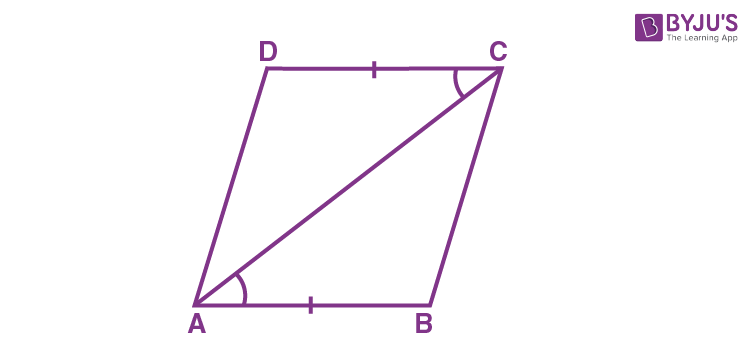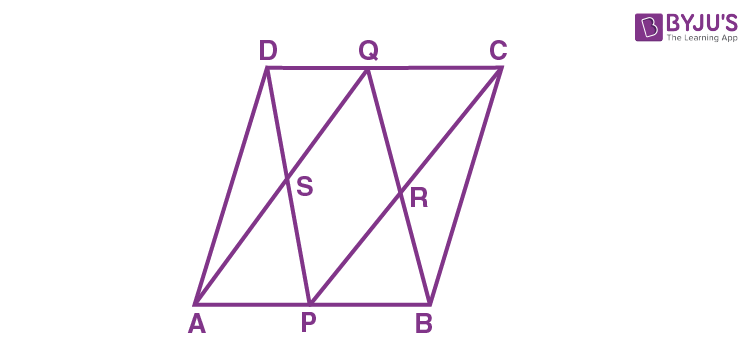# Another Condition for a Quadrilateral to be a Parallelogram

In Geometry, we have learned different types of polygons, such as triangle, quadrilateral, pentagons and so on. Based on the number of sides, polygons are classified into different types. A polygon with four sides is called a quadrilateral. Some of the examples of quadrilaterals are square, rectangle, rhombus, parallelogram, etc. We are quite familiar with the important properties of these quadrilaterals. Now, we are going to have a discussion about another condition for a quadrilateral to be a parallelogram with the help of an example.

## Another Condition for a Quadrilateral to be a Parallelogram – Theorem

We are familiar with some of the important properties of parallelogram, such as:

• The opposite side of a parallelogram are parallel and congruent
• The opposite angles of a parallelogram are equal
• Diagonals bisects each other
• Consecutive angles are supplementary, and so on.

Now, we are going to discuss yet another least required condition for a quadrilateral to be a parallelogram.

Theorem: A quadrilateral is a parallelogram if a pair of opposite sides is equal and parallel.In a quadrilateral ABCD, the side AB is equal to the side DC.

(i.e) AB = CD

Also, the side AB is parallel to the DC.

(i.e) AB || CD.

Now, draw the diagonal AC.

We know that a diagonal of a parallelogram divides the parallelogram into two congruent triangles.

By using the Side-Angle-Side (SAS rule), we can prove that ∆ ABC ≅ ∆ CDA.

Hence, the side BC is parallel to AD. (i.e) BC || AD.

Therefore, ABCD is a parallelogram.

### Example

Now, let us take an example to understand the property of a parallelogram.

Question:

ABCD is a parallelogram. The points P and Q are the mid-points of opposite sides AB and CD. If AQ intersects DP at S and BQ intersects CP at R. Prove that APCQ, DPBQ, PSQR are parallelograms.Solution:

Since, AB is parallel to CD, we can say AP || QC ….(1)

Given that, AP = ½ (AB) and CQ = ½ (CD)

Also, AB = CD

2AP = 2CQ

Now, cancel out “2” on both sides, we get

AP = CQ ….(2)

From (1) and (2), we can conclude that the opposite sides are equal and parallel to each other.

Therefore, a quadrilateral APCQ is a parallelogram.

Similarly, we can prove that quadrilateral DPBQ is a parallelogram, as DQ = PB and DQ || PB.